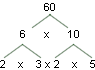www.batmath.it

A Glossary of Mathematical Terms - F

Face

Any of the flat sides of a polyhedron.

Factor (Divisor)

Each of the operands in a multiplication.
The multiplicative parts of a number: 1 is a factor of every number, 2 is a factor of every even number. Prime factors are factors that are prime numbers.

Factor treeA diagram used to determine the prime factors of a number:

Flip (Reflection)

A transformation of geometric figures that results in a mirror image of it.

Fraction

A number of the form a/b: a is the numerator, b is the denominator. If the numerator is bigger than the denominator the fraction is called proper, otherwise improper. Fractions are a way of representing rational numbers. A fraction is in the lowest or simplest form if the numerator and the denominator have non common factors: it is reduced to lowest terms.

Frequency diagram

A method used in statistics to record the collected data.

Function

A law that pairs all elements of a set with one and only one element of another set. Functions can be surjective (onto) injective, bijective (one-to-one). It is usually written in the form y = f(x) where x is the independent variable, y is the dependent variable. The first set is called the domain, the second set the codomain. The set of all possible values of the function is called the range (it is usually a subset of the codomain).

first published on september 01 2003 - last updated on september 01 2003